# FAMILIAR OPERATORS

The operation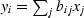is the multiplication of a matrix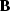by a vector.The adjoint operation is.The operation adjoint to multiplication by a matrix is multiplication by the transposed matrix (unless the matrix has complex elements, in which case we need the complex-conjugated transpose). The following pseudocode does matrix multiplication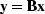and multiplication by the transpose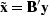:


if operator itself
then erase y
then erase x
do iy = 1, ny {
do ix = 1, nx {
if operator itself
y(iy) = y(iy) + b(iy,ix)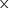x(ix)
x(ix) = x(ix) + b(iy,ix)y(iy)
}		 }


Notice that the bottom line'' in the program is that x and y are simply interchanged. The above example is a prototype of many to follow, so observe carefully the similarities and differences between the operation and its adjoint.

A formal subroutinefor matrix multiply and its adjoint is found below. The first step is a subroutine, adjnull(), for optionally erasing the output. With the option add=1, results accumulate like y=y+B*x.

subroutine adjnull( adj, add, x, nx,  y, ny )
real                          x( nx), y( ny )
do iy= 1, ny
y(iy) = 0.
else
do ix= 1, nx
x(ix) = 0.
return; end


The subroutine matmult() for matrix multiply and its adjoint exhibits the style that we will use repeatedly.

# matrix multiply and its adjoint
#
real                          bb(ny,nx), x(nx), y(ny)
do ix= 1, nx {
do iy= 1, ny {
y(iy) = y(iy) + bb(iy,ix) * x(ix)
else
x(ix) = x(ix) + bb(iy,ix) * y(iy)
}}
return; end


Sometimes a matrix operator reduces to a simple row or a column.

A row    is a summation operation.

A column is an impulse response.

If the inner loop of a matrix multiply ranges within a

row,    the operator is called sum or pull.

column, the operator is called spray or push.

A basic aspect of adjointness is that the adjoint of a row matrix operator is a column matrix operator. For example, the row operator [a,b]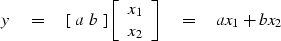(1)
has an adjoint that is two assignments: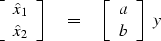(2)

 The adjoint of a sum of N terms is a collection of N assignments.